# CBSE Class 10 Maths Chapter 2 Polynomials Objective Questions

CBSE Class 10 Maths Chapter 2 Polynomials Objective Questions cover all the important concepts present in the chapter. It explains Polynomials and their applications in detail in this chapter. Students can learn about the division algorithm for polynomials of integers and also whether the zeros of quadratic polynomials are related to their coefficients from this chapter. As this is one of the important topics in Maths, it comes under the unit – Algebra. This CBSE Class 10 Maths Chapter 2 – Polynomials Objective Questions help students to get acquainted with solving MCQs.

Here, for the convenience of the students, we have compiled a list of topic-wise MCQs from this chapter. From the upcoming academic session, objective-type questions are expected to appear more often.

### List of Sub-Topics Covered in Chapter 2

Solving these CBSE Class 10 Maths Objective Questions will help the students to get a proper foundation in the subject. These MCQs are compiled in this article for students to download and practise so that they get acquainted with answering the objective type of questions. Meanwhile, see here the list of sub-topics we have covered in this chapter:

2.1 Basics Revisited (2 MCQs from This Topic)

2.2 Graphical Representations (2 MCQs Covered from This Topic)

2.3 Visualisation of a Polynomial (2 MCQs from This Topic)

2.4 Zeros of a Polynomial (3 MCQs from This Topic)

2.5 Factorisation of polynomials (3 MCQs from This Topic)

2.6 Relationship between Zeros and Coefficient (2 MCQs from The Topic)

2.7 Division Algorithm (2 MCQs Covered from This Topic)

2.8 Algebraic Identities (3 MCQs from This Topic)

## Download Free CBSE Class 10 Maths Chapter 2 – Polynomials Objective Questions PDF

### Basics Revisited

1. Write the coefficient of  x2 in each of the following.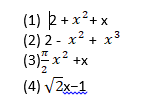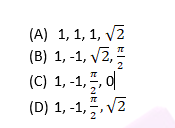Answer: C. 1, -1, π/2, 0

Solution: The constant multiplied by x2 is the coefficient of x2

(1) 2 + x2 + x → coefficient of x2 = 1

(2) 2 – x2 + x3 → coefficient of x2 = -1

(3) (π/2) x2 +x → coefficient of x= π/2

(4) √2 x−1 → coefficient of x2 = 0

1. The polynomial p(x) = x−323 is a ___.
1. Constant Polynomial
2. Cubic Polynomial
4. Linear Polynomial

Solution: The polynomial of degree one is called a linear polynomial.

Therefore, x−323 is a linear polynomial.

### Graphical Representations

1. Three curves, i.e. a) y = x2b) y = x4c) y = x6, are depicted in the graph shown below. Which of the polynomials does graph 3 represent?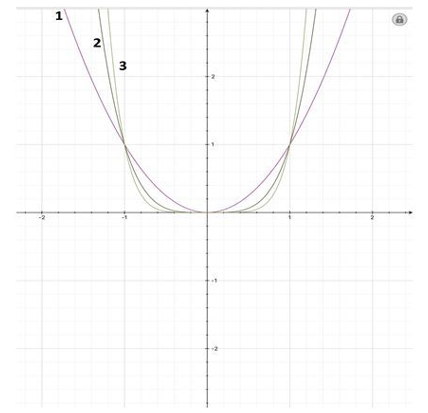1. y = x4
2. y = x6
3. y = x2
4. Cannot be determined

Solution: Consider the polynomial where n is a positive even integer.

As the value of n increases, then the curve goes closer to the positive y-axis.

Thus, graph 3 represents the polynomial x6

1. Three curves, i.e.,

a) Y = −x2

b) y = −x3

c) y = −x7

are depicted in the following graph and are numbered from 1 to 3.

Identify the correct relation.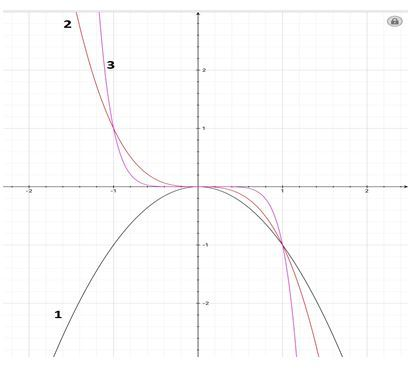1. (a)-(1) , (b)-(2), (c)-(3)
2. (a)-(3) , (b)-(2), (c)-(1)
3. (a)-(1) , (b)-(3), (c)-(2)
4. (a)-(2) , (b)-(3), (c)-(1)

Solutions: When a polynomial is of the form y = −xn, the graph of the polynomial is the mirror image of the graph of the polynomial y = xn.

Also, when the value of n increases, the graph draws closer to the y-axis.

Thus, graph 1 represents y = −x2, graph 2 represents y = −x3 and graph 3 represents y = −x7

### Visualisation of a Polynomial

1. If x = 2,y = −1, then the value of x2+4xy+4y2 is
1. 2
2. -1
3. 1
4. 0

Solution: Substituting the values,

X2+4xy+4y2

= (2)2 + 4(2) (-1) + 4(-1)2

= 4−8+4=0

1. According to the graph below, the product of the zeroes of the polynomial will be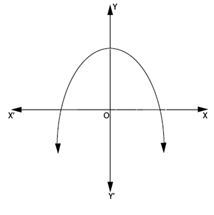1. Cannot be determined
2. Zero
3. Negative
4. Positive

Solution: One of the zeros of the polynomial lies on the positive x-axis. Thus, the abscissa or the x -coordinate, which is the corresponding zero, is positive.

The other zero lies on the negative x-axis. Thus, the abscissa or x -coordinate, which is the corresponding zero, is negative.

Thus, the product of zeroes is going to be positive negative = negative.

### Zeroes of a Polynomial

1. The number of polynomials having 3 and 7 as zeroes are
1. More than 3
2. 3
3. 2
4. 1

Solution: (x-3)A(X-7)B, where a and b can take any natural number values.

Hence, infinite possibilities.

1. If α, β and γ are the zeros of the polynomial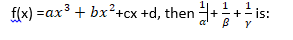1. – (b/a)
2. – (c/d)
3. a/d
4. c/d

Solutions: If α, β and γ are the zeros of the polynomial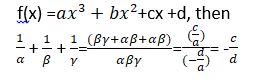1. If α, β are the zeros of the polynomial, x2-px +36 and α2 + β2 = 9, then what is the value of p?
1. 6
2. 3
3. 9
4. 8

Solution: Here a = 1, b = -p, c = 36.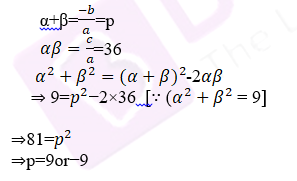### Factorisation of Polynomials

1. What is the factorisation of 2x2−7x−15?
1. (x+5) (2x-3)
2. (x+3) (2x-5)
3. (x-5) (2x+3)
4. (x-3) (2x-5)

Solution: Find two numbers such that their product is -30 and the sum is -7.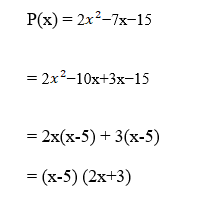1. What is the factorisation of x2 −5x+6?
1. (x+5) (x-3)
2. (x-6) (x+1)
3. (x-1) (x+5)
4. (x-2) (x-3)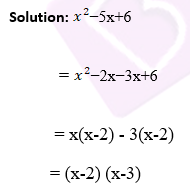1. Which among the following options is one of the factors of x2+ x/6+ 1/6?
1. 3x +1
2. 2x + 1
3. X – (1/5)
4. X- (1/2)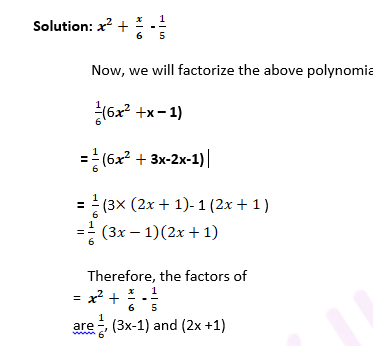### Relationship between Zeroes and Coefficients

1. Find the sum  and product of roots  for the given polynomial: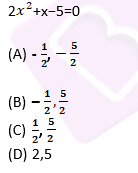Solution: We know that, for a quadratic equation

ax2 + bx+ c = 0 sum of roots = α+β & product of roots = αβ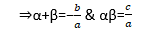Comparing 2x2+x−5=0 with ax2 + bx+ c=0, we get

a=2, b=1, c=−5

⇒α+β= – (1/2)

⇒αβ= – (5/2)

1. If p, q & r are the zeroes of a cubic polynomial ax3+bx2+cx+d, then what will be p+q+r?
1. c/a
2. b/a
3. –(c/a)
4. –(b/a)

Solution: We know that for a cubic polynomial ax3+bx2+cx+d Sum of zeroes = (b/a)

Therefore, p+q+r= – (b/a).

### Division Algorithm

1. In the division algorithm, when should one stop the division process?

1. When the remainder is zero.

2. When the degree of the remainder is less than the degree of the divisor.

3. When the degree of the quotient is less than the degree of the divisor.

1. Statements 1 and 2 are correct
2. Statements 2 and 3 are correct
3. Statements 1 and 3 are correct
4. Only statement 3 is correct

Answer: (A) Statements 1 and 2 are correct

Solution: We stop the division process when either the remainder is zero, or its degree is less than the degree of the divisor.

1. If the remainder when x3+2x2+kx+3 is divided by x-3 is 21, find the zeroes of x3+2x2+kx−18.
1. -2, 3, 3
2. -3, 2, 3
3. -3, -2,3
4. -3,-3, 2

Solution: P (3) = 48 + 3k = 21

⇒ K = -9

Hence, x3+2x2−9x+3= (x−3) x Quotient + 21

⇒x3+2x2−9x−18 =9x−3) x Quotient

Quotient = (x3+2x2−9x−18) / (x-3)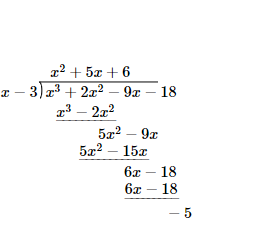Factorising the quotient, x2+ 5x +6= x2+ 3x + 2x +6 = x(x+3) +2(x+3) =(x+2) (x+3)

Hence, the factors of x3+2x2−9x−18 are x−3, x+2 and x+3

⇒ the zeroes are -3,-2, 3.

### Algebraic Identities

1. If x+x-1 = 10, (x≠0), then what will be x2+x-2?
1. 100
2. 10
3. 98
4. 102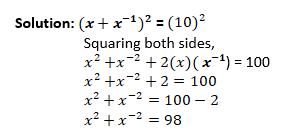1. f α and β are the zeros of the polynomial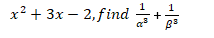1. –(45/8)
2. 45/8
3. -(8)/ 45
4. 8/45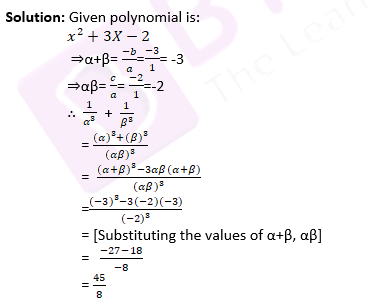1. What term should be added to a2+2ab to make it a perfect square?
1. 2ab
2. a2
3. b2

Solution: To make (a2+2ab) a perfect square, b2 is to be added.

So (a2+2ab+b2) will become a perfect square using the identity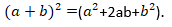Here, we have compiled 19 questions from Chapter 2 Polynomials of the CBSE Class 10 Maths in the downloadable PDF link that we have given in the article above. Also, find some extra CBSE Class 10 Maths Chapter 2 MCQs here.

## CBSE Class 10 Maths Chapter 2 Extra MCQs

1. Given that one zero of the quadratic polynomial x² + 3x + k is 2,  what is the value of k?
(a) -10
(b) 10
(c) 5
(d) -5

2. Given that the zeroes of the quadratic polynomial x2 + (a + 1) x + b are 2 and -3, what are a and b?
(a) a = 5, b = -1
(b) a = -7, b = -1
(c) a = 2, b = -6
(d) a – 0, b = -6

3. The number of zeroes that a linear polynomial has/have is _____.
(a) 1
(b) 2
(c) 0
(d) 3

Keep learning and stay tuned to BYJU’S to get the latest news on the CBSE exam along with study materials, sample question papers, marking schemes, and more.